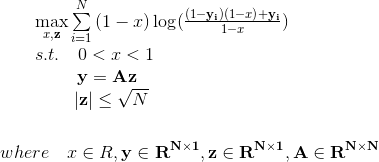# 'cvx_bound' is 'Inf' but 'cvx_optval' is caculated as '9.667'

My optimization problem is acceptable for CVX, but a wrong solution will be computed. It should be noted that ‘cvx_bound’ is ‘Inf’ but ‘cvx_optval’ is caculated as ‘9.667’ which may be reason for wrong solution. The optimization problem is formulated as:clear ,clc
N=3;
A=[ 2.3133 2.0017 2.2737; 2.5309 2.9248 2.6868; 3.6302 3.3929 3.6934];
cvx_begin
variable x
variable z(N)
y=A*z;
maximize(sum(-rel_entr((1-x),(1-y) * (1-x)+y))/log(2))
0 <= x <= 1
norm(z)<=sqrt(N)
cvx_end

Thanks very much.

The code you show should not be accepted by CVX. In particular, the (`1-y) * (1-x)` term in the 2nd argument of `rel_entr` violates CVX’s DVP rules.

Moreover, according to my calculations, the Hessian of `(1-x)*log(((1-x)*(1-y)+y)/(1-x))` is indefinite. Therefore, the objective function is neither convex nor concave, and therefore can not be reformulated to be acepeted by CVX.

If you have a run in which your program was accepted and CVX produced an optimal solution, please show all the output.

Please start a new MATLAB session. Then copy and paste your program into that new session and run it. Show us all the output. Also show the output from running `cvx_version` .

Please copy and paste, using Preformatted text icon, that MATLAB session into a post. Do not post an image. I am still trying to figure out how your program could execute without an error message from CVX in your `maximize` statement.

output：
x = 3.4676e-10
y =
6.5933
8.1366
10.7211
z =
1.0002
0.9798
1.0196

``````A =
2.3133    2.0017    2.2737
2.5309    2.9248    2.6868
3.6302    3.3929    3.6934

cvx_bound =   Inf
cvx_cputime =    1.3281
cvx_optval =    9.6674
cvx_slvitr =    35
cvx_slvtol =   1.7120e-09
cvx_status =Solved
``````

cvx_version：
---------------------------------------------------------------------------
CVX: Software for Disciplined Convex Programming ©2014 CVX Research
Version 3.0beta, Build 1175 (1326ef2) Mon Nov 23 14:29:34 2015
---------------------------------------------------------------------------

result display：
Successive approximation method to be employed.
SDPT3 will be called several times to refine the solution.
Original size: 18 variables, 10 equality constraints
3 exponentials add 21 variables, 12 equality constraints
-----------------------------------------------------------------
Cones | Errors |
Mov/Act | Centering Exp cone Poly cone | Status
--------±--------------------------------±--------
3/ 3 | 2.645e+00 4.207e-01 0.000e+00 | Solved
3/ 3 | 1.783e-01 2.090e-03 0.000e+00 | Solved
3/ 3 | 5.132e-03 1.719e-06 0.000e+00 | Solved
0/ 0 | 0.000e+00 0.000e+00 0.000e+00 | Solved
-----------------------------------------------------------------
Status: Solved
Optimal value (cvx_optval): +9.66742

I think this program must have been accepted by CVX due to a bug in CVX 3.0beta. CVX 3.0beta does extend he DCP rules and allow some programs which CVX 2,1 does not, but this program should not be accepted. I have no idea what problem CVX 3.0beta actually is submitting to the solver, but youy should consider any answer provided to be wrong.

I recommend you switch to CVX 2.,1 and not use CVX 3.0beta. Given your interest in problems involving log, rel_entr , etc. I suggest you install CVXQUAD and follow the instructions at CVXQUAD: How to use CVXQUAD's Pade Approximant instead of CVX's unreliable Successive Approximation for GP mode, log, exp, entr, rel_entr, kl_div, log_det, det_rootn, exponential cone. CVXQUAD's Quantum (Matrix) Entropy & Matrix Log related functions

Edit: I see you used CvX 3.0beta Build 1175, which is not the most recent build of 3.0beta. I still recommend that you not use CVX 3.0beta, but if you do, at least change to 3.0beta Build 1183, which is the most recent 3.0beta vuild.

Edit2: I ran this program under CVX 30beta Build 1183, and duplicated your results. I then specified `cvx_solver scs`, which utilized scs’ native exponential cone capability, and got the same result (to several decimal places). So apparently CVX 3.0beta is formulating the same wrong problem both for its successive approximation method and for utilization of the native exponential cone capability of scs.

Thank you so much for your advice! I will try to use CVX 2.,1 and rethink the optimization problem.

A little birdie told me the optimal solution to your non-convex problem above is

optimal objective = 4.9010

optimal x = 0.4322

optimal z = [1.0001 0.9799 1.0196]’

Note that the `norm(z) <= sqrt(N)` constraint is active (satisfied with equality) at the optimum.

I will not assess whether the problem you provided is a good real-world model of whatever you are trying to apply it to, but it is a “valid” mathematical optimization problem which has a solution. if that is the problem you actually want to solve, I recommend you use something other than CVX.